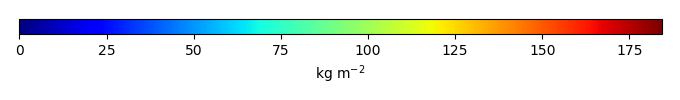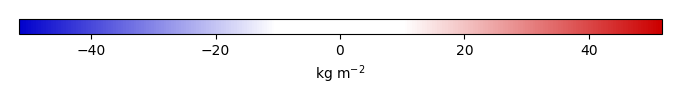# Mean State

Period Mean (original grids) [Pg]
Model Period Mean (intersection) [Pg]
Model Period Mean (complement) [Pg]
Benchmark Period Mean (intersection) [Pg]
Benchmark Period Mean (complement) [Pg]
Bias [kg m-2]
Bias Score 
Spatial Distribution Score 
Overall Score 
Benchmark [-] 76.5
CRUNCEPv7 [-] 248. 232. 16.7 76.1 0.362 57.1 0.24 0.075 0.16
GSWP3v1 [-] 101. 90.3 10.0 76.1 0.362 6.17 0.53 0.30 0.42
WATCH [-] 127. 116. 10.9 76.1 0.362 16.0 0.46 0.25 0.36
Period Mean (original grids) [Pg]
Model Period Mean (intersection) [Pg]
Model Period Mean (complement) [Pg]
Benchmark Period Mean (intersection) [Pg]
Benchmark Period Mean (complement) [Pg]
Bias [kg m-2]
Bias Score 
Spatial Distribution Score 
Overall Score 
Benchmark [-] 113.
CRUNCEPv7 [-] 294. 194. 94.1 113. 0.00185 16.5 0.52 0.55 0.54
GSWP3v1 [-] 133. 70.5 59.6 113. 0.00185 -9.00 0.56 0.83 0.69
WATCH [-] 137. 87.7 45.7 113. 0.00185 -5.38 0.64 0.75 0.70
Period Mean (original grids) [Pg]
Model Period Mean (intersection) [Pg]
Model Period Mean (complement) [Pg]
Benchmark Period Mean (intersection) [Pg]
Benchmark Period Mean (complement) [Pg]
Bias [kg m-2]
Bias Score 
Spatial Distribution Score 
Overall Score 
Benchmark [-] 12.2
CRUNCEPv7 [-] 122. 13.3 113. 12.2 0.00 1.52 0.53 0.26 0.40
GSWP3v1 [-] 91.8 5.31 88.9 12.2 0.00 -6.98 0.55 0.82 0.68
WATCH [-] 115. 15.0 102. 12.2 0.00 2.54 0.62 0.51 0.56
Period Mean (original grids) [Pg]
Model Period Mean (intersection) [Pg]
Model Period Mean (complement) [Pg]
Benchmark Period Mean (intersection) [Pg]
Benchmark Period Mean (complement) [Pg]
Bias [kg m-2]
Bias Score 
Spatial Distribution Score 
Overall Score 
Benchmark [-] 97.3
CRUNCEPv7 [-] 254. 242. 14.9 97.2 0.0996 46.5 0.41 0.10 0.26
GSWP3v1 [-] 119. 110. 10.0 97.2 0.0996 4.55 0.54 0.39 0.47
WATCH [-] 84.2 76.8 8.49 97.2 0.0996 -6.18 0.58 0.64 0.61
Period Mean (original grids) [Pg]
Model Period Mean (intersection) [Pg]
Model Period Mean (complement) [Pg]
Benchmark Period Mean (intersection) [Pg]
Benchmark Period Mean (complement) [Pg]
Bias [kg m-2]
Bias Score 
Spatial Distribution Score 
Overall Score 
Benchmark [-] 5.12
CRUNCEPv7 [-] 67.9 4.90 62.0 5.12 0.00 -0.930 0.70 0.89 0.80
GSWP3v1 [-] 44.2 3.46 40.1 5.12 0.00 -6.30 0.64 0.62 0.63
WATCH [-] 33.7 2.09 31.1 5.12 0.00 -11.3 0.54 0.49 0.51
Period Mean (original grids) [Pg]
Model Period Mean (intersection) [Pg]
Model Period Mean (complement) [Pg]
Benchmark Period Mean (intersection) [Pg]
Benchmark Period Mean (complement) [Pg]
Bias [kg m-2]
Bias Score 
Spatial Distribution Score 
Overall Score 
Benchmark [-] 475.
CRUNCEPv7 [-] 1.92e+03 1.08e+03 836. 474. 1.51 27.6 0.43 0.19 0.31
GSWP3v1 [-] 1.06e+03 433. 622. 474. 1.51 -1.41 0.53 0.64 0.59
WATCH [-] 1.09e+03 478. 608. 474. 1.51 0.756 0.56 0.50 0.53
Period Mean (original grids) [Pg]
Model Period Mean (intersection) [Pg]
Model Period Mean (complement) [Pg]
Benchmark Period Mean (intersection) [Pg]
Benchmark Period Mean (complement) [Pg]
Bias [kg m-2]
Bias Score 
Spatial Distribution Score 
Overall Score 
Benchmark [-] 116.
CRUNCEPv7 [-] 326. 313. 14.0 116. 0.373 34.5 0.41 0.15 0.28
GSWP3v1 [-] 123. 115. 7.60 116. 0.373 0.749 0.49 0.62 0.56
WATCH [-] 154. 145. 8.77 116. 0.373 6.01 0.51 0.30 0.40
Period Mean (original grids) [Pg]
Model Period Mean (intersection) [Pg]
Model Period Mean (complement) [Pg]
Benchmark Period Mean (intersection) [Pg]
Benchmark Period Mean (complement) [Pg]
Bias [kg m-2]
Bias Score 
Spatial Distribution Score 
Overall Score 
Benchmark [-] 0.0131
CRUNCEPv7 [-] 42.8 0.0285 42.1 0.0131 0.00 6.98 0.31 3.3e-20 0.15
GSWP3v1 [-] 32.2 0.0178 31.5 0.0131 0.00 2.19 0.69 2.5e-23 0.35
WATCH [-] 31.5 0.0166 30.9 0.0131 0.00 1.63 0.76 3.0e-22 0.38
Period Mean (original grids) [Pg]
Model Period Mean (intersection) [Pg]
Model Period Mean (complement) [Pg]
Benchmark Period Mean (intersection) [Pg]
Benchmark Period Mean (complement) [Pg]
Bias [kg m-2]
Bias Score 
Spatial Distribution Score 
Overall Score 
Benchmark [-] 46.1
CRUNCEPv7 [-] 145. 81.7 61.9 46.1 0.00725 16.6 0.54 0.68 0.61
GSWP3v1 [-] 75.2 36.0 39.5 46.1 0.00725 -4.90 0.56 0.58 0.57
WATCH [-] 68.6 32.4 36.6 46.1 0.00725 -6.67 0.58 0.49 0.54
Period Mean (original grids) [Pg]
Model Period Mean (intersection) [Pg]
Model Period Mean (complement) [Pg]
Benchmark Period Mean (intersection) [Pg]
Benchmark Period Mean (complement) [Pg]
Bias [kg m-2]
Bias Score 
Spatial Distribution Score 
Overall Score 
Benchmark [-] 0.232
CRUNCEPv7 [-] 12.9 0.398 13.2 0.232 0.00 1.94 0.60 0.71 0.65
GSWP3v1 [-] 11.5 0.289 11.9 0.232 0.00 0.143 0.53 0.81 0.67
WATCH [-] 12.5 0.397 12.8 0.232 0.00 1.89 0.53 0.78 0.65

# Temporally integrated period mean

BENCHMARK MEANMODEL MEANBIASBIAS SCORESPATIAL TAYLOR DIAGRAMMODEL COLORS# Spatially integrated regional mean

MODEL COLORS# All Models

BenchmarkCRUNCEPv7GSWP3v1WATCH# Data Information

Approach: I just read the data from the original data file and converted the latitude arrange from original 90N->90S to 90S->90N. The unit was convert from original 0.1 KgC/m2 to KgC/m2

Temporal resolution: yearly

General information: This product was derived from Northern Circumpolar Soil Carbon Database V2.2 (NCSCDv22) in top 1m.

Spatial resolution: 0.5x0.5 degree